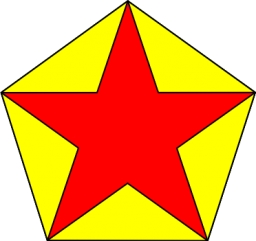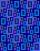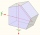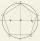# Pentagon

Calculate the area of a regular pentagon, which diagonal is u=16.

Correct result:

S =  168.23

#### Solution:We would be pleased if you find an error in the word problem, spelling mistakes, or inaccuracies and send it to us. Thank you!Tips to related online calculators

#### You need to know the following knowledge to solve this word math problem:

We encourage you to watch this tutorial video on this math problem:

## Next similar math problems:

• Regular 5-gonCalculate the area of the regular pentagon with side 7 cm.
• Diagonals of pentagonCalculate the diagonal length of the regular pentagon: a) inscribed in a circle of radius 12dm; b) a circumscribed circle with a radius of 12dm.
• PentagonCalculate the length of side, circumference and area of a regular pentagon, which is inscribed in a circle with radius r = 6 cm.Calculate the content of a regular 15-sides polygon inscribed in a circle with radius r = 4. Express the result to two decimal places.
• Hexagon ACalculate area of regular hexagon inscribed in circle with radius r=9 cm.
• DiagonalDiagonal of the rectangle has length 46.4 cm. Angle between diagonal and londer sife of rectangle is 22°. Calculate area of the rectangle.
• Hexagon ABCDEFIn the regular hexagon ABCDEF, the diagonal AE has a length 8cm. Calculate the circumference and the hexagon area.
• Inscribed and described circleFind the radii of a circle inscribed and circumscribed by a regular pentagon whose side measures 3 cm.
• SAS triangleThe triangle has two sides long 7 and 19 and included angle 36°. Calculate area of this triangle.
• Regular n-gonWhich regular polygon have a radius of circumscribed circle r = 10 cm and the radius of inscribed circle p = 9.962 cm?
• Triangle ABC v2Area of the triangle is 12 cm square. Angle ACB = 30º , AC = (x + 2) cm, BC = x cm. Calculate the value of x.
• PentagonWithin a regular pentagon ABCDE point P is such that the triangle is equilateral ABP. How big is the angle BCP? Make a sketch.
• Nine-gonCalculate the perimeter of a regular nonagon (9-gon) inscribed in a circle with a radius 13 cm.
• Pentagonal prismThe regular pentagonal prism is 10 cm high. The radius of the circle of the described base is 8 cm. Calculate the volume and surface area of the prism.
• Right triangleCalculate the missing side b and interior angles, perimeter, and area of ​​a right triangle if a=10 cm and hypotenuse c = 16 cm.
• Triangle SASCalculate the triangle area and perimeter, if the two sides are 51 cm and 110 cm long and angle them clamped is 130 °.
• DecagonCalculate the area and circumference of the regular decagon when its radius of a circle circumscribing is R = 1m